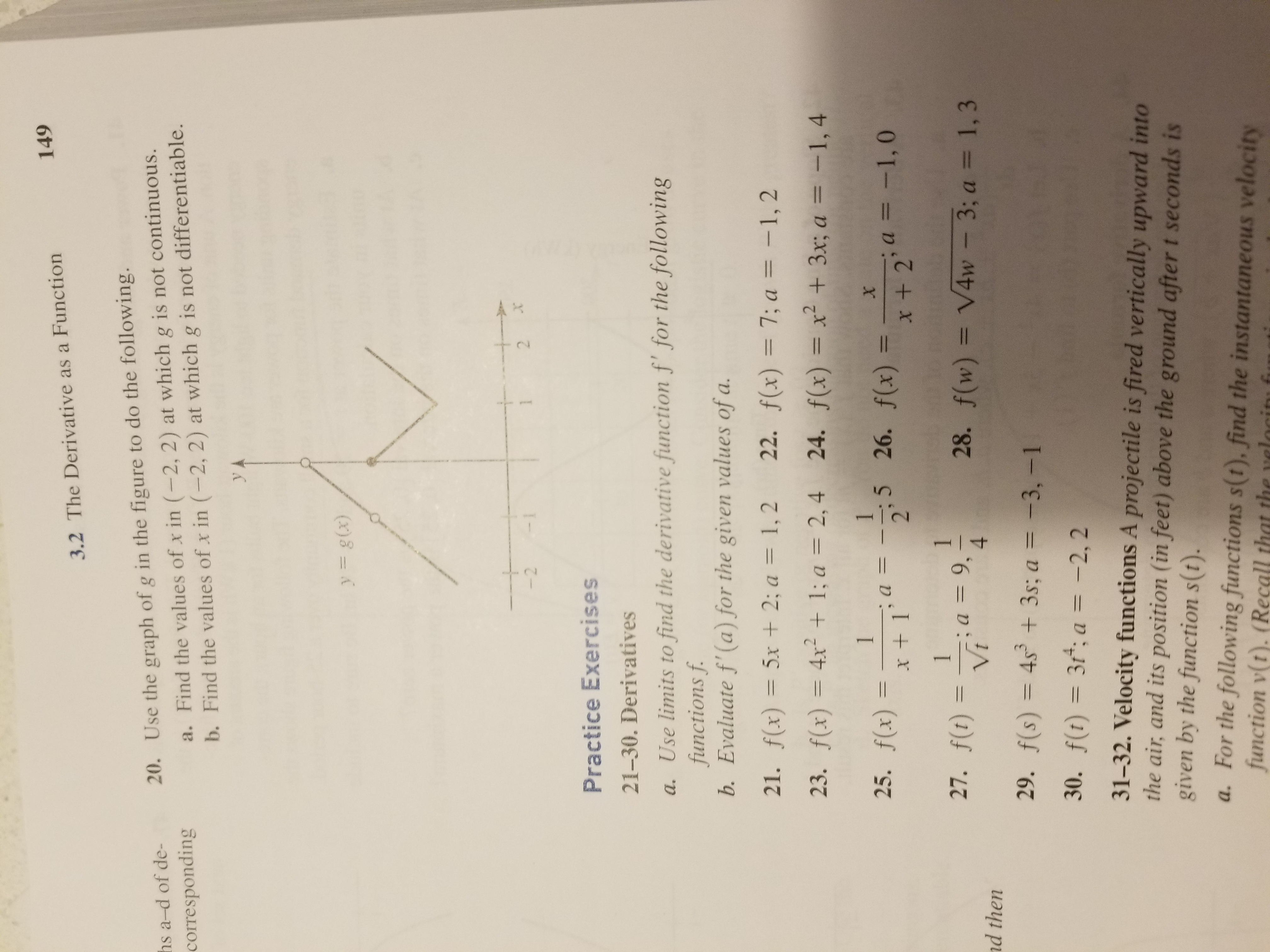# 1493.2 The Derivative as a FunctionUse the graph of g in the figure to do the following.a. Find the values of x in (-2, 2) at which g is not continuous.b. Find the values of x in (-2,2) at which g is not differentiable.20.hsa-d of de-correspondingy= g(x)Practice Exercises21-30. Derivativesa. Use limits to find the derivative function f' for the followingfunctions fb. Evaluate f'(a) for the given values of a21. f(x)=5x + 2; a= 1,2 22. f(x)=7;a=-1,223. f(x) = 4x2 + 1; a = 2,4 24. f(x) = x2 + 3x; a =-1.425. f(x) =-1, a =ー2,5 26. f(x) =-; a =-1,0x +x+ 227. f(r) =-lt; a = 9,4f(w)-V4w-3; a = 1,328.d then29,f(s) = 4s3 + 3s; a =-3,-130.f(t)=3°; a=-2, 231-32. Velocity functions A projectile is fired vertically upward intothe air, and its position (in feet) above the ground after t seconds isgiven by the function s(t).a. For the following functions s(t), find the instantaneous velocityfunction v(t). (Recall that the velacit f

Question
128 views

How would you solve #25help_outlineImage Transcriptionclose149 3.2 The Derivative as a Function Use the graph of g in the figure to do the following. a. Find the values of x in (-2, 2) at which g is not continuous. b. Find the values of x in (-2,2) at which g is not differentiable. 20. hs a-d of de- corresponding y= g(x) Practice Exercises 21-30. Derivatives a. Use limits to find the derivative function f' for the following functions f b. Evaluate f'(a) for the given values of a 21. f(x)=5x + 2; a= 1,2 22. f(x)=7;a=-1,2 23. f(x) = 4x2 + 1; a = 2,4 24. f(x) = x2 + 3x; a =-1.4 25. f(x) =-1, a =ー2,5 26. f(x) =-; a =-1,0 x + x+ 2 27. f(r) =-lt; a = 9,4 f(w)-V4w-3; a = 1,3 28. d then 29, f(s) = 4s3 + 3s; a =-3,-1 30. f(t)=3°; a=-2, 2 31-32. Velocity functions A projectile is fired vertically upward into the air, and its position (in feet) above the ground after t seconds is given by the function s(t). a. For the following functions s(t), find the instantaneous velocity function v(t). (Recall that the velacit f fullscreen
check_circle

Step 1

(a) To find f'(x) , starting from the definition of derivative as a limit; (b) using (a) , to calculate the derivatives at the given values of x.

Step 2

The derivative f'(x) for a general function f(x) is defined in terms of the limit as shown.

Step 3

Apply this to the given function f(x) and go through t...

### Want to see the full answer?

See Solution

#### Want to see this answer and more?

Solutions are written by subject experts who are available 24/7. Questions are typically answered within 1 hour.*

See Solution
*Response times may vary by subject and question.
Tagged in

### Derivative